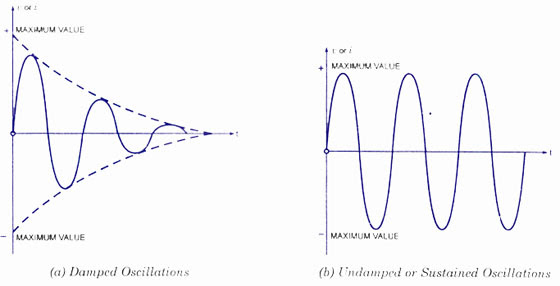## Audio

[Audio][slideshow]

This article has two sections: One discusses criteria for selecting an oscillator while other one discusses various types of oscillations.

1. Selection of an Oscillator

2. Types of Oscillations

## Criteria for Selection of an Oscillator

Here are the parameters that are to be noted while selecting an oscillator for a particular application.

1. Frequency Range. The oscillator selected for a particular application should be ca­pable of supplying an output signal whose upper and lower frequency limits exceed those required by the application.
2. Power and/or Voltage. The oscillator selected for a particular application should be capable of generating the pertinent quantity with a magnitude large enough to meet the requirement.
3. Accuracy and Dial Resolution. The accuracy of an oscillator specifies how closely the output frequency corresponds to the frequency indicated on the dial of the in­strument. Dial resolution indicates to what percentage of the output frequency value the dial setting can be read.
4. Amplitude and Frequency Stability. The amplitude stability is a measure of an oscillator’s ability of maintaining constant voltage amplitude with variations in the output signal frequency. Frequency stability determines how closely the oscillator maintains a constant fre­quency over a given time period. Sometimes the frequency stability is included in the accuracy specifications of the oscillator.
5. Waveform Distortion.This quantity is a measure of how closely the output wave­form of the oscillator resembles a pure sinusoidal signal. Sometimes the oscillator is employed as a source in a test used for measuring the tendency of a circuit to distort a sinusoidal signal. In such tests, the distortion caused by the oscillator should be much less than the anticipated distortion because of the circuit under test.
6. Output Impedance. The output impedance of an oscillator specifies the impedance value of the load which must be connected to it for maximum power transfer. It is very important that the output impedance of the oscillator be equal to the char­acteristic impedance of the system to which it is to be connected.

## Damped and Undamped Oscillations

### Damped Oscillations:

Damped oscillations is clearly shown in the figure (a) given below. In such a case, during each oscillation, some energy is lost due to electrical losses (I2R). The amplitude of the oscillation will be reduced to zero as no compensating ar­rangement for the electrical losses is provided. The only parameters that will remain unchanged are the frequency or time period. They will change only according to the circuit parameters.Damped and Umdamped Oscillations

### Undamped Oscillations:

As shown in figure (b), undamped oscillations have constant amplitude oscillations. In the harmonic oscillation equation, the exponential factor e_Rt/2L must become unity. That is, the value of the dissipation component in the circuit, R should be zero. If its value is negative, the amplitude goes on increasing with time t. If its value is positive, the amplitude decreases with time t.

In order to obtain undamped oscillations in any physical circuit, the positive value of the dissipation component, R must be neutralized with a negative resistance. The correct amount of undamped oscillations will be obtained only if the correct amount of energy is supplied to overcome the losses at the right time in each cycle. The resulting “undamped oscillations” are called sustained oscillations. Such sustained oscillations or continuous waves are required to be produced by the electronic oscillator circuits.

### Listed below are articles related to this one – Take a look.

FREQUENCY STABILITY OF OSCILLATORS

BASIC OSCILLATORY CIRCUITS

OSCILLATOR OPERATION

LC OSCILLATORS AND TYPES

AUDIO OSCILLATORS

[Lights][grids]